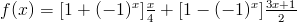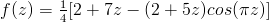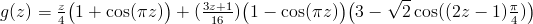Blog > The Collatz Conjecture as a Fractal

## The Collatz Conjecture as a Fractal

June 20th, 2009

The Collatz conjecture (or hailstone problem or 3n+1 problem) is a problem that is so simple to state that grade-schoolers can understand it, yet has been approached from an innumerable variety of angles and has resisted mathematicians for decades now. The problem is as follows:

Pick a positive integer. If it’s even then divide it by 2. If it’s odd, multiply it by 3 and add 1. Now apply this procedure to the result. Repeat. Will you always eventually hit 1 if you continue in this way?

For example, if you start with 11 then you multiply by 3 and add 1 to get 34, divide by 2 to get 17, and continuing similarly gets you the rest of the sequence: 52, 26, 13, 40, 20, 10, 5, 16, 8, 4, 2, 1. Before you go trying to find a counterexample to the conjecture, know that it has been verified by computer search for all starting numbers up to 20 × 258 ≈ 5.764 × 1018. The way that we are going to look at this problem today is the “pretty” approach; we’re going to extend the Collatz conjecture over the complex plane and look at the fractal defined by its iteration.

Define the Collatz function f(x) as follows:Take a moment or two to convinve yourself that if x is a positive integer, then f(x) is the next number after x in its Collatz sequence (e.g., f(11) = 34, f(34) = 17, and so on). To extend this function to the real numbers, simply recall that (-1)x = cos(πx). In fact, this gets us an extension to the complex numbers at the same time, and after some simplification we arrive at:Well hey, that’s a holomorphic function so it has a notion of a Julia set and we can study the fractal that its iterates induce. Indeed, we can obtain the following image pretty easily with standard fractal-generation software:

The fractal is located on the complex plane, and the horizontal line through the middle of the image is the real line. Black regions are regions in which the orbit of that number is bounded, while other colours indicate that the orbit of that number is unbounded (notice the large region of bounded numbers around z = 0). The big “spikes” that occur along the real line are, as we would expect, located at the integers (the image above is wide enough that you can see the spikes at z = -2, -1, 1, and 2). Instead of proceeding with the Collatz function as I have defined it, I’m going to introduce a modified Collatz function g(z) as follows:Observe that this function, like f(z), always maps natural numbers to terms that appear later in their Collatz sequence (e.g., g(11) = 17, g(17) = 13). The benefit of this function is that it has the additional property that g(1) = 1. That is, 1 is a fixed point of g(z), whereas it is part of a period-3 cycle of f(z). The fractal induced by g(z) is:

I originally moved to using g(z) instead of f(z) because the plot of the f(z) fractal from earlier indicated to me that there may be a ball of black (i.e., boundedness) of non-zero radius around each of the integers, but proving this for f(z) seemed to be quite difficult (as we would expect, since it would basically imply half of the Collatz conjecture). Somewhat strangely, even though there appear to be balls around the integers in the fractal induced by f(z), these balls vanish in the fractal induced by g(z). The image to the right is a close-up of the above fractal (the point of convergence is z = 1).

Nonetheless, the real line still seems to behave reasonably nicely under the action of g(z); it’s not difficult to prove that the fractal contains the real line segment [0,N] for some large number N that is similar in magnitude to the least M such that the conjecture is true for all n ≤ M (known to be at least 5×1018 or so, as mentioned earlier). However, many (non-natural number) points in that interval do not converge to 1.

So what now? If the Collatz conjecture is true, then z = 1 is the unique natural number fixed point of g(z), yet there seem to be points arbitrarily close to z = 1 that don’t even converge under iteration of g(z). Why are there smaller spikes between the integers in both of these fractals, and where are the spikes centered? Does the restriction of the Collatz function to the numbers at the center of those spikes have any simple interpretation? Who knows, I’ll explore some more in the future. Until then, enjoy some pretty pictures.

Tags:
1. June 27th, 2009 at 23:04 | #1

Wiki actually mentions this approach – http://en.wikipedia.org/wiki/Collatz_conjecture#Iterating_on_real_or_complex_numbers – one would assume that there’s some actual research into it already? Or at least some speculation. Although I *still* don’t know complex analysis, but your rephrasing to g(z) seems interesting.

2. June 28th, 2009 at 12:19 | #2

Ha, go figure I make a post about something that’s described a fair amount in a wiki page that I even linked to. Unfortunately it doesn’t seem to have a reference to anything like this so I’m not sure what exactly is known. Oh well, at least it gave me an excuse to make some pretty pictures.

PS. How do you not know any complex analysis yet — aren’t you at McGill (ie. the school the scares the bejesus out of me because they introduce you to abstract algebra in first year)? What year are you in now by the way (I should really know this, I apologize)?

3. June 28th, 2009 at 16:02 | #3

Nah, it’s okay, no one really knows what year I’m in! Let’s say I’m about 18 credits off from graduation, and I have been in university for 2 full years. I’ll probably go over the minimum credit requirement and finish in the spring anyway (I’m told it’s okay because I have no electives >.>) And, well, I don’t know complex analysis because I have a somewhat reduced math requirement because of my econ major (double-majoring, if you recall). It’s not technically mandatory, although I might take it this fall or winter anyway. Also, I’ve focused more on the umm… applied, I suppose, aspects of math (since I’m sort of thinking of going into finance, perhaps). So, I’ve taken courses in stochastic processes, non-linear dynamics, and graph theory, but still no complex analysis.

Also, while wiki doesn’t cite sources, Google scholar provides a few results, although I haven’t exactly read through them. Very little is new under the sun, I suppose (especially in well-researched areas ;)). http://scholar.google.ca/scholar?&q=Collatz+fractal

4. August 6th, 2010 at 14:30 | #4

Your pretty pictures have all but disappeared. 🙁

5. August 6th, 2010 at 21:50 | #5

@Michael Blackburn – Thanks for letting me know. I moved my site to a new server yesterday and some random things like that got lost in the switch. Fixed now 🙂

6. August 10th, 2010 at 19:45 | #6

Did you notice that in your z=4 pic there are 8 “spikes. And in the z=8 pic there are exactly 16 spikes. And in the Z=16 pic there are exactly 32 spikes. ??? makes me wonder if every z=2^n pic will have exactly 2^(n+1) spikes. Neat project!

7. June 28th, 2011 at 07:09 | #7

I like to know what software you had used to generate fractal surface of f(z) and g(z )at z= one integer value. Moreover is it possible to get fractal image of 3x + 1 or 3z+1 mapping at discrete values.

8. July 1st, 2011 at 10:11 | #8

@Rammohan.R – It was a while ago now, but I believe I used Ultra Fractal.

9. January 6th, 2012 at 10:37 | #9
10. March 25th, 2012 at 15:19 | #10

Great article! This morning I was thinking about whether anyone had thought about examining the Collatz conjecture with fractals and I found your blog. Keep up the good work 🙂

11. April 12th, 2012 at 10:46 | #11

Heh. I’ve just started writing my new blog about collatz problem and I came on this site by case. And I’ve just seen that your blog looks almost the same like mine and whats more you have writing about quite the same topics like me. Interesting correlation…

12. July 15th, 2013 at 15:25 | #12

Very neat article. I wasn’t expecting the google query “collatz conjecture extended to reals” to be so fruitful.

Do you know (or intuit) whether or not the convergent set is fully connected? It *looks* like there are islands in the images for z=4, z=8, and z=16, but is it possible that their unconnected appearance is a rendering artefact?

13. October 1st, 2021 at 00:11 | #13

Nice! I watched Veritasium’s YouTube video about Collatz conjecture and immediately Googled for its fractal emanation, and found your article. Thank you for doing this all those years ago!

14. October 9th, 2021 at 22:30 | #14

The Collatz functions show up only as “Collatz function”, “Complex Collatz function”, and “Modified Collatz function”. Could this be changed to show the actual functions? Thanks. I would really like to understand your article better.

15. January 25th, 2022 at 12:40 | #15

@John, the link to the image file containing the function definition is broken. (It seems to be using an old host name.) Here’s the correct link:

It would be great if these image links could be fixed.

16. January 28th, 2022 at 12:50 | #16

Glad I stumbled upon this. I’ve been staring at the numbers using basic math over the last few months and came to the conclusion that the 3n+1 conjecture is a fractal. I have in mind the overall shape that unfolds as the numbers increase. Created shortcuts to get from higher numbers to one and found patterns which prompted me to find the overall pattern.

Looks like a fractal!

17. February 23rd, 2022 at 16:08 | #17

@Neil Mayhew – Thanks, fixed now.

1. June 4th, 2011 at 03:47 | #1
2. October 29th, 2012 at 07:58 | #2
3. October 6th, 2013 at 13:57 | #3
4. November 11th, 2015 at 08:43 | #4